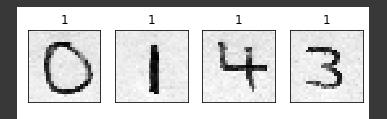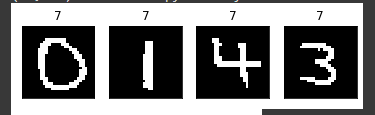# Expanding MNIST digit recognizer outside the MNIST test dataset

I followed chapter 4 to understand how to build a handwritten digit classifier.
Using a CNN model i was able to train it and get ~100% accuracy.

However, i tried fetching a picture of handwriting digits from Google and test my model on it, and it failed miserably (100% of the times)

Here is my model

``````features_to_track  = 3
model = torch.nn.Sequential(
# first cnn layer with a 3x3 filter, thus it outputs a tensor of size 26.
# since the image is grayscale, the in_channels is 1.
torch.nn.Conv2d(in_channels=1, out_channels=features_to_track,kernel_size=3,stride=1),
torch.nn.ReLU(),

# max poolin with stride of 2, therefor it downsample by x2 each dim of the image
torch.nn.MaxPool2d(2,2),

torch.nn.Conv2d(in_channels=features_to_track, out_channels=2,kernel_size=3,stride=1),
torch.nn.ReLU(),

torch.nn.Conv2d(in_channels=2, out_channels=1,kernel_size=3,stride=1),
torch.nn.ReLU(),

torch.nn.Flatten(),

torch.nn.Linear(in_features=81, out_features=20),
torch.nn.ReLU(),
torch.nn.Linear(in_features=20, out_features=10),
torch.nn.Sigmoid(),
)
loss_fn= torch.nn.CrossEntropyLoss()
optim = torch.optim.SGD(params=model.parameters(), lr=0.001)

epochs = 50

for _ in range(epochs):
# predict
predictions = model(train_img_batch)

# loss
loss = loss_fn(predictions, train_label_batch)

# backward
loss.backward()

# step (update params)
optim.step()
``````

I’m testing my model with this image, where i cropped each digit, and build this function:

``````import cv2 as cv
from skimage import io

url = 'https://user-images.githubusercontent.com/40036314/48663507-57567a00-eab7-11e8-822e-ac80eace819b.jpg'
image_2 = cv.cvtColor(image, cv.COLOR_BGR2GRAY)

digits = [
image_2[208:340, 560:660],
image_2[170:320, 120:230],
image_2[200:340, 454:550],
image_2[180:320, 225:360]
]
resized = [cv.resize(d, (28,28)) for d in digits]
tensors = [torch.tensor(d).unsqueeze(0)/255 for d in resized]

fig = plt.figure()
for i, t in enumerate(tensors):
plt.subplot(1,4,i+1)
plt.imshow(t.squeeze(), cmap='gray', interpolation='none')
plt.title(predict(t))
plt.xticks([])
plt.yticks([])
fig
``````(the number above the digit pic is what my model predicted it’ll be)

if i change the number of epochs, then nothing changes here, except the prediction, but always it predicts the same thing for all digits.

Any idea what’s wrong with my model? how can i debug those things?

It could be several things but looking at the image you reference, the background pixels are not zero but the MNIST data set background is 0 so I have a feeling this wouldn’t work very well. Try zeroing out the background pixels and see if that does better.

Yes, i figured this could be an issue, so i filtered the gray values, but still same problem.
i think it has to do with the model architecture, but can’t point how exactly.Are you sure you’re not overfitting?

that’s very plausible, but how can i test that the model is overfitting vs. if the architecture is not robust? (im a noob at this, apologies if it’s a sily question)
ps. i tried changing the model in many different ways (adding another cnn/ keeping one cnn, as well as the in and out channels per cnn), however kept getting the wrong results with my external image

You have to look at your training loss vs. your validation loss. It’s in the bookAnd it’s not a silly question at all.

From what you’ve written, it seems like you need a more structured approach to figuring out what’s wrong instead of just trying to change stuff to see what happens. This is hard to learn and hard to teach. But start with checking if you’re overfitting.

Did you solve the problem?
I tried transfer learning but it predicts the same thing for custom images.

I resized the custom images to (28,28) its working now.1 Like

Nice!